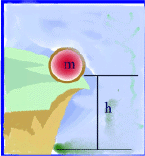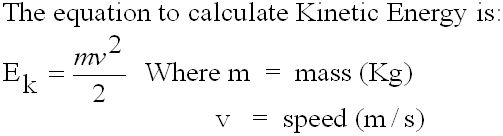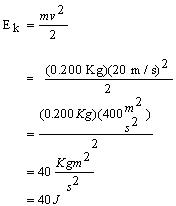#### Energy

Energy is defined as the ability to do work.  Energy can take many forms.  In mechanics the two most common forms of energy are Kinetic Energy and Potential Energy.  The general symbol for all energy forms is E.  Energy is measured in Joules (J).

#### Potential Energy

Potential Energy is the energy stored in an object when it has been lifted off the ground.  The symbol for Potential Energy is EPIn the figure above, an object with mass m (in kilograms) is lifted up to a height of h meters above the ground, against gravity.  This object will have stored gravitational potential energy EP

To calculate this stored (or sometimes called "latent" or "cached") energy we use the equation below:

EP= m x g x h

The units of Potential Energy, are the same as the unit of work. Work and energy are both calculated in (J), "Joules".

Example 1:
In the picture above, the ball on the cliff has a mass of 300 g and the cliff is 20 meters above the ground.  What is the potential energy of the ball?

Given:

m = 300

h = 20 m

g = 9.8 m/s2 or 9.8 N/Kg

g = 0.300 Kg

Find:

EP

Solution:

EP = m x g x h

= (0.300 Kg)((9.8 N/Kg)(20 m)

= 58.8 Nm

Therefore the mass will have 58.8 J of stored (Potential Energy) at the top of the cliff.

#### Kinetic Energy

Kinetic Energy is the energy of a moving object.  The symbol for Kinetic Energy is Ek

The kinetic energy of an object depends on the mass (m) of the object and on the speed (v) of the object.Example 2:

A 200 g soccer ball is moving with a speed of 20.0 m/s.  Calculate its kinetic energy.

Given:

m = 200 g = 0.200 Kg
v = 20 m/s

Find:

Ek

Solution:Therefore the soccer ball has a Kinetic Energy 40 J